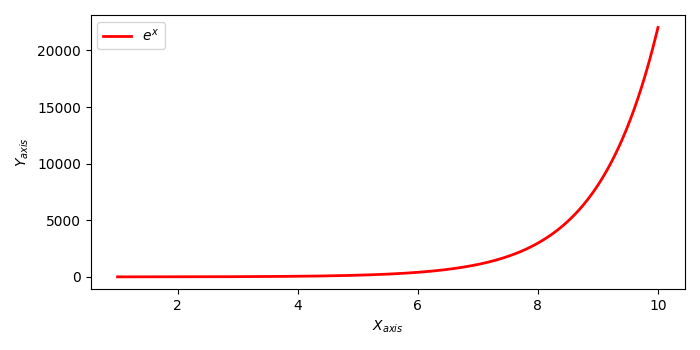# How to write text in subscript in the axis labels and the legend using Matplotlib?

To write text in subscript in the axis labels and the legend, we can take the following steps −

• Create x and y data points using NumPy.

• Plot x and y data points with a super subscript texts label.

• Use xlabel and ylabel with subscripts in the text.

• Use the legend() method to place a legend in the plot.

• To display the figure, use the show() method.

## Example

import numpy as np
import matplotlib.pyplot as plt
plt.rcParams["figure.figsize"] = [7.00, 3.50]
plt.rcParams["figure.autolayout"] = True
x = np.linspace(1, 10, 1000)
y = np.exp(x)
plt.plot(x, y, label=r'$e^x$', c="red", lw=2)
plt.xlabel("$X_{axis}$")
plt.ylabel("$Y_{axis}$")
plt.legend(loc='upper left')
plt.show()

## Output